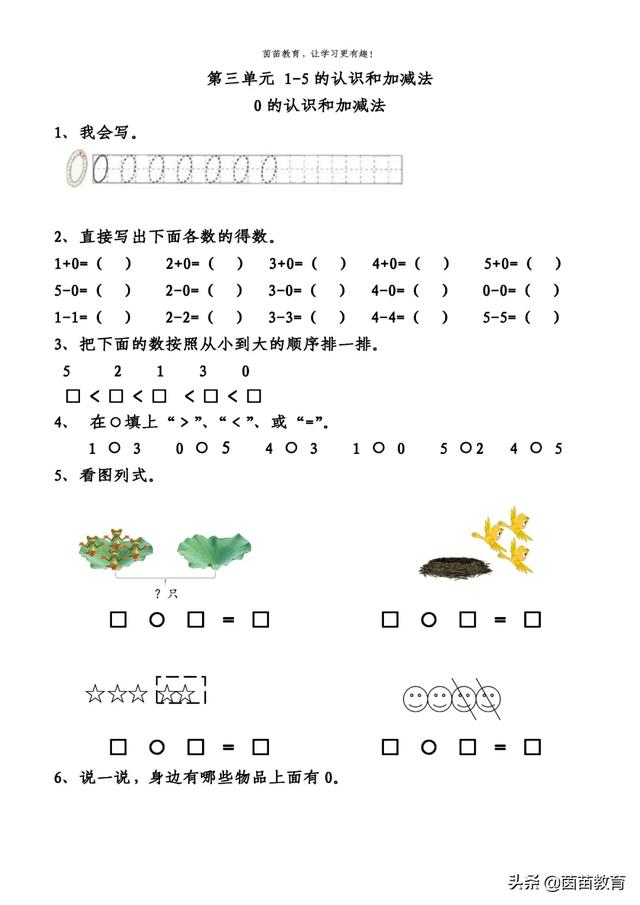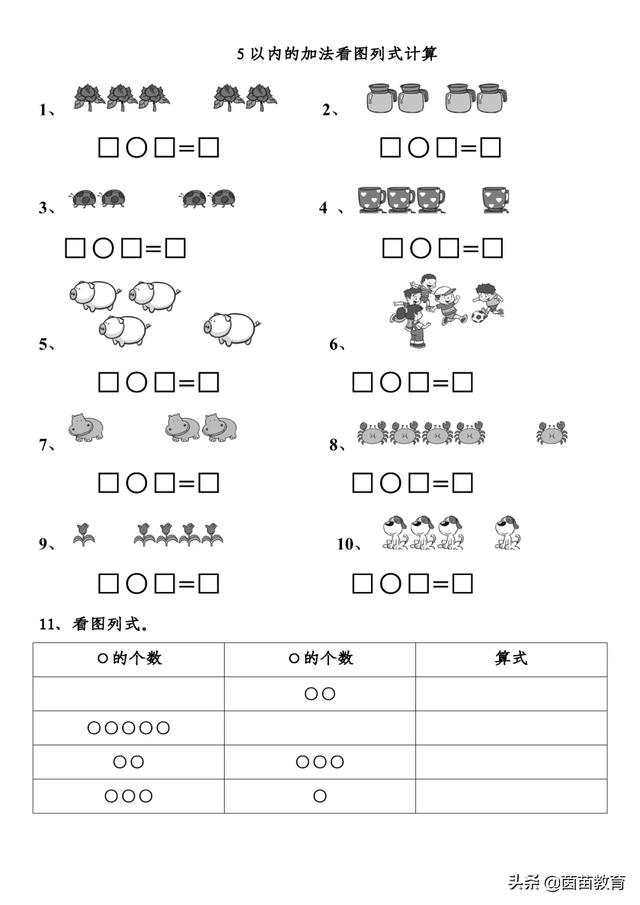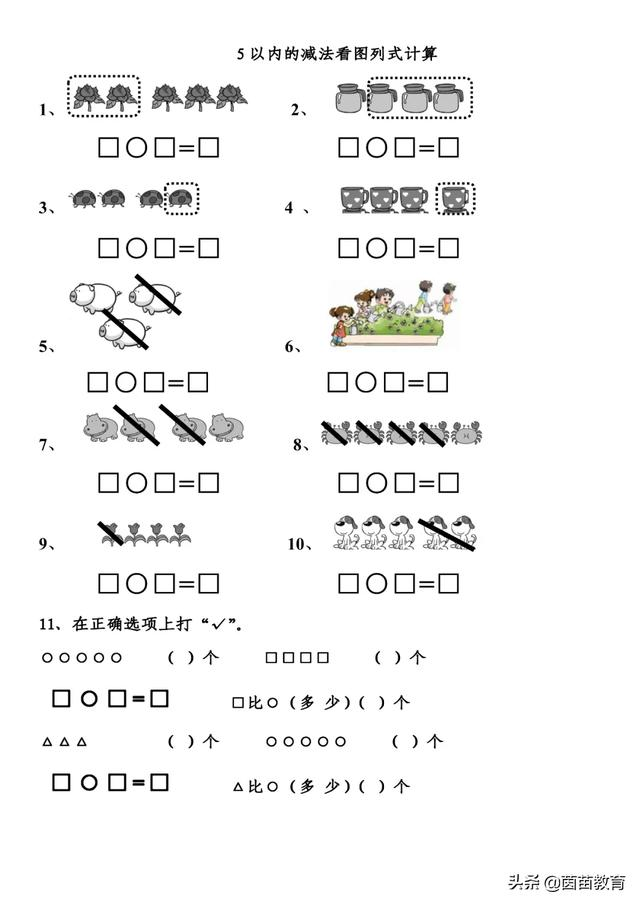# 3.3一年级上册数学《0-5的加减法》同步练习，附答案

2021-01-04 35 ℃1、我会写。

2、直接写出下面各数的得数。

1+0=（0） 2+0=（2） 3+0=（3） 4+0=（4） 5+0=（5）

5-0=（5） 2-0=（2） 3-0=（3） 4-0=（4） 0-0=（0）

1-1=（0） 2-2=（0） 3-3=（0） 4-4=（0） 5-5=（0）

3、把下面的数按照从小到大的顺序排一排。

5 2 1 3 0

0<1<2<3<5

4、 在○填上“＞”、“＜”、或“=”。

1 < 3 0 < 5 4> 3 1 > 0 5 >2 4<5

5、看图列式。

4+0=4 3-3=0

5-2=3 4-2=2

6、说一说，身边有哪些物品上面有0。1、3+2=5

2、2+2=4

3、2+2=4

4、3+1=4

5、3+1=4

6、4+1=5

7、1+2=3

8、4+1=5

9、1+4=5

10、3+1=4

11、

○的个数 ○的个数 算式

○○ 0+2=2

○○○○○ 5+0=5

○○ ○○○ 2+3=5

○○○ ○ 3+1=41、5-2=3

2、4-3=2

3、4-1=3

4、4-1=3

5、3-2=1

6、5-2=3

7、4-2=2

8、5-4=1

9、4-1=3

10、5-2=3

11、在正确选项上打“√”。

○○○○○ （5）个

□□□□ （4）个

5-4=1

△△△ （3）个

○○○○○ （5）个

5-3=2

△比○（少）（2）个

（此处已添加圈子卡片，请到今日头条客户端查看）